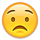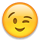## Forum Stats

• 3,733,814 Users
• 2,246,824 Discussions
• 7,856,883 Comments

Discussions

#### Howdy, Stranger!

It looks like you're new here. If you want to get involved, click one of these buttons!

# function to get the interest rate

hi;
iam trying to develop a function to return te rate of a loan knowing the original ammount, monthly payment, term.

basically what i need is the same as rate function in excel but i cant find the matematical function to calculate the rate

thanks

orestes
Tagged:

## Answers

• Check this link.

561331

Regards

Raj
• I found this formula on http://www.fonerbooks.com/solving.htm
the guy borrowed 100,000 for the mortgage and is making monthly payments of \$830.33 for 15 years
looks like to solve for the interest they take the equation
P / M = [ i(1 + i)^n ] / [ (1 + i)^n - 1]

and make a guess at the interest rate and keep incrementing until the two sides match (or are close)

anyway I did in sql starting with and interated .01% interest rate and and incrementing by .01% for
10000 iterations and I ordered by the smallest difference between the two sides of the equation
and came up with 5.75% which I believe is correct

```WITH variables AS (    SELECT 100000 M,
830.33 P,
180 n,
LEVEL * .0001 i
FROM DUAL
CONNECT BY LEVEL <= 10000)
SELECT i * 100 || '%' interest_rate,
P / M,
( (i / 12) * POWER ( (1 + (i / 12)), n))
/ (POWER ( (1 + (i / 12)), n) - 1)
FROM variables
ORDER BY ABS (
(P / M)
- ( ( (i / 12) * POWER ( (1 + (i / 12)), n))
/ (POWER ( (1 + (i / 12)), n) - 1)))```
```INTEREST_RATE	P/M	((I/12)*POWER((1+(I/12)),N))/(POWER((1+(I/12)),N)-1)
5.75%	0.0083033	0.00830410087019666
5.74%	0.0083033	0.00829874710327995
5.76%	0.0083033	0.00830945655888689
5.73%	0.0083033	0.00829339525864719
5.77%	0.0083033	0.00831481416883952
5.72%	0.0083033	0.00828804533680813
5.78%	0.0083033	0.00832017369954276
5.71%	0.0083033	0.00828269733827184
5.79%	0.0083033	0.00832553515048413```
you could probably make this better so it doesn't have to do all the iterations if you use a recursive with or the model clause
and stop when the difference between the two sides of the equation is less then some number.

Edited by: pollywog on Feb 18, 2011 3:11 PM
• You don't need 10000 iterationsAlmost 40 years ago I was taught to rewrite the formula as (speeding up convergence):
<font face="courier" size = "3"><b> i := PM * (1 - 1 / POWER(1 + i,n12)) </b></font> (a monthly interest rate gets computed)
computing separately:
<font face="courier" size = "3"><b> n12 = 12 * n </b></font> and <font face="courier" size = "3"><b> PM = P / M </b></font> using PM as the first approximation for <font face="courier" size = "3"><b> i </b></font> on the right side
iterating until successive approximation differr only after some chosen decimal position (less than 40 iterations should get you 10 decimal positions)
dont forget to multiply by 12 the solution obtained to get the year rateI don't have database access to provide a tested solution and haven't heard of our model man for quite a time (I don't feel like providing a model solution without testing either)

Regards

Etbin

Edited by: Etbin on 19.2.2011 8:17

PM introduced (to get a single multiplication in each iteration instead of a multiplication and a division)

Edited by: Etbin on 19.2.2011 8:27
• ```declare
m       number := 100000;
p       number := 830.33;
n       number := 15;
n12     number := 12 * n;
pm      number := p / m;
i       number := pm;
old_i   number := 2 * pm;
ret_val number;
cnt     pls_integer := 0;
begin
--  while old_i > i                    /* to compute to maximum precision */
while old_i - i > 5 * power(10,-11)  /* to achieve 10 digits precision */
loop
cnt := cnt + 1;
old_i := i;
i := pm * (1 - 1 / power(1 + i,n12));
if cnt > 500 then
exit;
end if;
dbms_output.put_line(to_char(cnt)||' '||to_char(old_i)||' '||to_char(i)||' '||to_char(old_i - i));
end loop;
ret_val := 12 * i;
dbms_output.put_line(to_char(cnt)||' iterations to return '||to_char(ret_val));
end;```
This discussion has been closed.